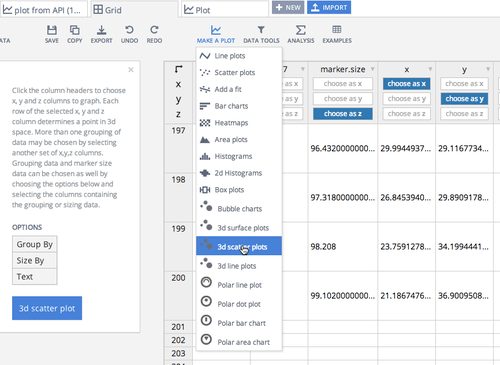Want to share your content on R-bloggers? click here if you have a blog, or here if you don't.

by Matt Sundquist
Plotly, co-founder

Plotly is a platform for data analysis, graphing, and collaboration. You can use ggplot2, Plotly's R API, and Plotly's web app to make and share interactive plots. Now, you can you can also make 3D plots. Immediately below are a few examples of 3D plots.  In this post we will show how to make 3D plots with ggplot2 and Plotly's R API.First, let's convert a ggplot2 tile plane into a Plotly graph, then convert it to a 3D plot. You can copy and paste this code and use a test username and key, or sign up for an account and generate your own.

```install.packages("devtools")  # so we can install from github
library("devtools")
install_github("ropensci/plotly")  # plotly is part of ropensci
library(plotly)

py <- plotly(username="r_user_guide", key="mw5isa4yqp")  # open plotly connection

pp <- function (n,r=4) {
x <- seq(-r*pi, r*pi, len=n)
df <- expand.grid(x=x, y=x)
df\$r <- sqrt(df\$x^2 + df\$y^2)
df\$z <- cos(df\$r^2)*exp(-df\$r/6)
df
}
p <- ggplot(pp(20), aes(x=x,y=y))

p <- p + geom_tile(aes(fill=z))

py\$ggplotly(p)```

We return a URL: plot.ly/~r_user_guide/83/y-vs-x/. The URL hosts the interactive plot, rendered with D3.js, a JavaScript visualization library. Each plot stores the data, and code to reproduce a plot with MATLAB, Python, R, Julia, and JavaScript.We can export or embed plots in Shiny Apps, knitr, Slidify, blogs, and in an iframe, as we're doing below.

We'll now style, share, and change to 3D in the web app. Press the "Fork and Edit" button to get started in the GUI. The web app runs online and is free, so you won't need to install or download anything.Below is our edited plot. Go to the plot and press " View full-size graph" to really dig in, or go straight to a full-screen version: plot.ly/~MattSundquist/2260.embed. Try clicking, holding, and toggling to flip, drag, and zoom. Press the icons in the upper right-hand corner to change modes.

We can also use qplot and edit the plot in the GUI. Assuming you ran the code above:

```qplot(x, y, data=pp(100), geom="tile", fill=z)

py\$ggplotly()```

We can also make 3D plots from a grid of imported Excel, Dropbox, Google Drive, or pasted data. Or combine data from another plot. Privacy settings are like Google Drive and GitHub: you control if plots are public or private, you own your data and can add collaborators, or you can run Plotly on-premise.To create a 3D plot directly from R, we can use Plotly's R API. For example, try this code to make a surface plot. Plotly also supports 3D line and scatter plots.

```data <- list(
list(
z = matrix(c(1, 20, 30, 50, 1, 20, 1, 60, 80, 30, 30, 60, 1, -10, 20), nrow=3, ncol=5),
x = c("Monday", "Tuesday", "Wednesday", "Thursday", "Friday"),
y = c("Morning", "Afternoon", "Evening"),
type = "surface"
)
)
response <- py\$plotly(data, kwargs=list(filename="3D surface", fileopt="overwrite"))
url <- response\$url  # returns plot URL```

Editing in the GUI offers options for editing the lighting, lines that appear when hovering, colors in the scene and grid lines, and axis titles.The plot, as well as code to reproduce our new version, is also available at the URL: plot.ly/~MattSundquist/2263.

3D plots can be useful for showing three-dimensional spaces like world, lakes, or mountains. You can also plot a random walk, the Lorenz attractor, functions, and stock volatility. We welcome your feedback, thoughts, and suggestions. We're at feedback at plot.ly and @plotlygraphs. Happy plotting!